# Time and Work Problems With Solutions

Time and Work Problems With Solutions, deals with various concepts which are as under:-

• Finding time taken by one person to finish a piece of work
• Time to finish work by more efficient person
• Time to finish work by 2 person when individual time given
• Time taken by 3 person to finish work
• Three person – Time taken by third to finish work
• Time taken by two taps to fill a cistern together
• Two taps – One filling and one emptying the cistern

#### Time and Work Problems With Solutions – Finding time taken by one person to finish a piece of work

Question 1

X and Y together can do a piece of work in 8 days, while Y can finish the same work, working alone in 12 days. Find the number of days in which X alone can finish the work?

Explanation:

Work done by X in one day = Work done by ( X + Y ) in one day – Work done by Y in one day

Given:

Time taken by ( X + Y ) to complete the work = 8 days

Time taken by Y to complete the work = 12 days

Work done by ( X + Y ) in one day = 1/8 units

Work done by Y in one day = 1/12 units

Work done by X in one day = Work done by ( X + Y ) in one day – Work done by Y in one day

= 1/8 – 1/12

= (3 – 2)/24

= 1/24

Since, Work to be completed and Days Taken have inverse relationship, We will just take an inverse of units produced in one day to know the time taken.

Time taken by X to complete the work = 24 days

#### Time and Work Problems With Solutions – Time to finish work by more efficient person

Question 2

X can do a piece of work in 26 days. Y is 30 % more efficient than X. Find the number of days in which Y can finish a piece of work?

Explanation:

Time taken by X to finish a piece of work = 26 days

Work done by X in one day = 1/26 units

Given:

Y is 30 % more efficient than X

i.e, Work done by Y in one day = Work done by X in one day + 30% of Work done by X in one day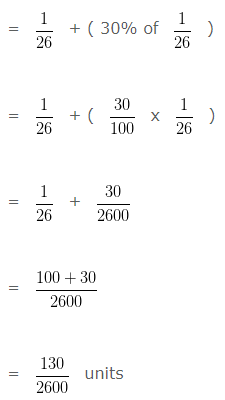Since, Work to be completed and Days Taken have inverse relationship, We will just take an inverse of units produced in one day to know the time taken.

Time taken by Y to finish a piece of work = 2600/130 days

= 20 days

#### Time and Work Problems With Solutions – Time to finish work by 2 person when individual time given

Question 3

X can finish a piece of work in 12 days and Y can do the same work in 24 days. Find the number of days in which total work can be completed if both of them work together?

Explanation:

Time taken by X to finish the piece of work = 12 days

Time taken by Y to finish the piece of work = 24 days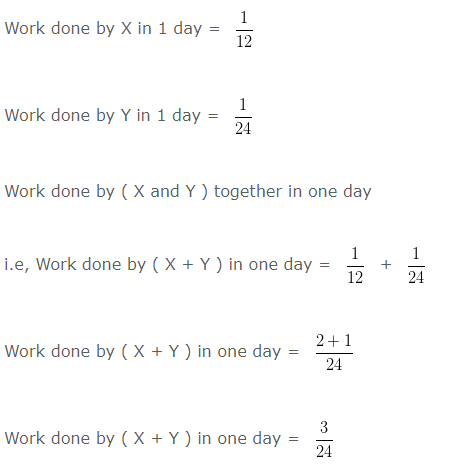then, Time taken by ( X + Y ) to complete the piece of work = 24/3 days = 8 days

#### Time and Work Problems With Solutions –  Time taken by 3 person to finish work

Question 4

A and B together can do a piece of work in 3 days ; B and C can do it in 6 days , while A and C can do it in 9 days . Find the number of days in which A , B and C can together finish the piece of work?

Explanation:

Time taken by ( A + B ) to finish a piece of work = 3 days

Time taken by ( B + C ) to finish a piece of work = 6 days

Time taken by ( A + C ) to finish a piece of work = 9 days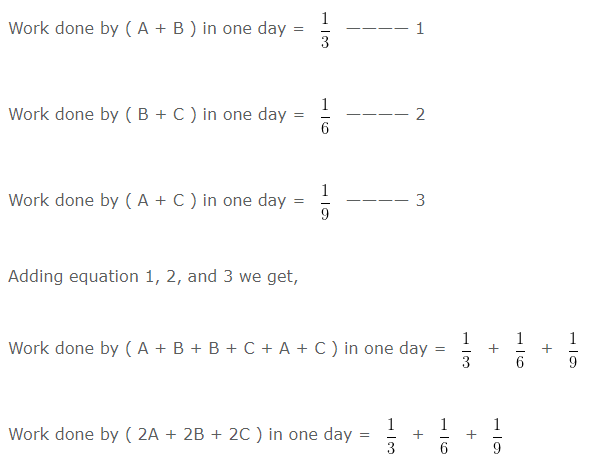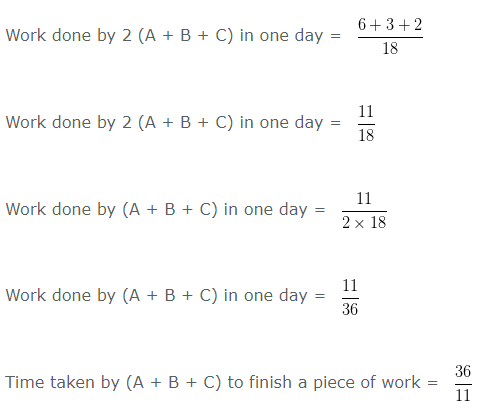]

#### Time taken by third person to finish work

Question 5

A , B and C can do a piece of work in 10 days. A and B can do it alone in 20 days and 30 days respectively. Find the number of days in which C alone can finish the work?

Explanation:

Time taken by ( A + B + C ) to finish a piece of work = 10 days

Time taken by A to finish a piece of work = 20 days

Time taken by B to finish a piece of work = 30 days

Work done by ( A + B + C ) in one day = 1/10 — Equation 1

Work done by A in one day = 1/20 — Equation 2

Work done by B in one day = 1/30 — Equation 3

Subtracting Equation 2 and Equation 3 from Equation 1

i.e, Equation 1 – Equation 2 – Equation 3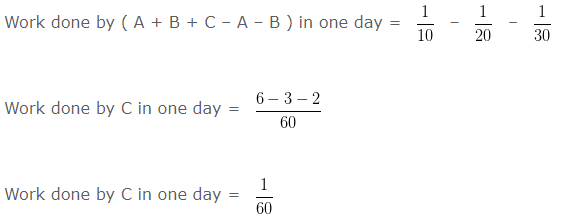Time taken by C to finish a piece of work = 60 days

#### Time and Work Problems With Solutions – Time taken by two taps to fill a cistern together

Question 6

Tap A and Tap B can fill a cistern in 5 hours and 7 hours respectively. If both the taps are opened simultaneously, then the cistern will be filled in?

Explanation:

Time taken by Tap A to fill the cistern = 5 hours

Time taken by Tap B to fill the cistern = 7 hours

Cistern filled by Tap A in one hour = 1/5

Cistern filled by Tap B in one hour = 1/7

Cistern filled by Tap ( A + B ) in one hour = 1/5 + 1/7

Cistern filled by Tap ( A + B ) in one hour = (7+5)/35

Cistern filled by Tap ( A + B ) in one hour = 12/35

Time taken by Tap A and B together to fill the cistern = 35/12

#### Two taps – One filling and one emptying the cistern

Question 7

Tap I can fill a cistern in 4 hours while tap II can empty the full cistern in 6 hours. If both the taps are opened, then the cistern will be filled in how many number of hours ?

Explanation:

Time taken by Tap I to fill the cistern = 4 hours

Time taken by Tap II to empty the full cistern = 6 hours

Cistern filled by Tap I in one hour = 1/4

Cistern empltied by Tap II in one hour = (-1)/6 ( tap II empties the cistern )

Cistern filled by Tap ( I + II ) in one hour = 1/4 + (-1)/6

Cistern filled by Tap ( I + II ) in one hour = 1/4 – 1/6

Cistern filled by Tap ( I + II ) in one hour = (3-2)/12

Cistern filled by Tap ( I + II ) in one hour = 1/12

Time taken by Tap I and Tap II to fill the cistern if they are opened simultaneously = 12 hours